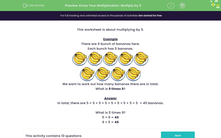# Know Your Multiplication: Multiply by 5

In this worksheet, students will learn to multiply by five.Key stage:  KS 1

Curriculum topic:   Number: Multiplication and Division

Curriculum subtopic:   Use Multiplication/Division Facts (2, 5, 10)

Popular topics:   Multiplication worksheets

Difficulty level:#### Worksheet Overview

This activity is about multiplying by 5.

Example

There are 9 bunches of bananas here.

Each bunch has 5 bananas.We want to work out how many bananas there are in total.

What are 9 lots of 5?

In total, there are 5 + 5 + 5 + 5 + 5 + 5 + 5 + 5 + 5  = 45 bananas.

What is 9 times 5?

9 x 5 = 45

5 x 9 = 45

Let's try another example.There are 6 hands above and each hand has 5 fingers (if you include the thumbs!)

We want to work out how many fingers there are in total.

What are 6 lots of 5?

In total, there are 5 + 5 + 5 + 5 + 5 + 5 = 30 fingers.

What is 6 times 5?

6 x 5 = 30

5 x 6 = 30

Are you ready to try some questions?### What is EdPlace?

We're your National Curriculum aligned online education content provider helping each child succeed in English, maths and science from year 1 to GCSE. With an EdPlace account you’ll be able to track and measure progress, helping each child achieve their best. We build confidence and attainment by personalising each child’s learning at a level that suits them.

Get started